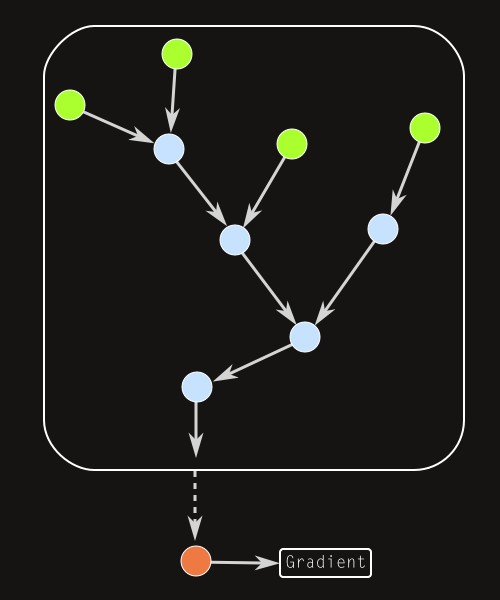# Algorithmic worlds

## -

Back to the information page

### About algorithmic worlds

Introduction
Algorithmic art
Pictorial algorithms
Ultra Fractal
Algorithmic worlds
Piling patterns
The structure
Pattern generators
Index operators
The piling operator
An example
Other modules

## The structure of the algorithm

The pictorial algorithms used to produce the works displayed here decompose into basic modules, ie. smaller algorithms. These are essentially of three types, as summarized in the table below: the pattern generators, the index operators, and a single module of the last type, the piling operator.

ModuleInputOutputAim
Pattern generator Pixel coordinates Index Creates a pattern
Index operator Index Index Modifies a pattern
Piling operator Pattern Index Piles scaled copies of a pattern

The pattern generators draw simple patterns. Practically, they read the coordinates of the pixel currently computed and use them to produce a value of the index (see the section Ultra Fractal).

The index operators are used to modify the visual aspect of the patterns. To this end, they transform the index produced by a pattern generator. They accept one or several values of the index as an input and perform some mathematical operations on it before they return it. They may also use the coordinates of the pixel to carry out their computations.

As a result, the general structure of the algorithms built out of the first two types of modules is a tree graph, like the one depicted in the white box in the diagram below. The arrows represent a value of the index transferred from one module to another. The leaves correspond to pattern generators (in green) while the nodes are index operators (in blue). The tree has a single root, so the corresponding algorithm is a pictorial algorithm: it returns a single value of the index given a value of the pixel coordinates, hence produces a pattern. A concrete example will be provided later.As we mentionned, to produce an algorithmic world, we want to pile copies of a given pattern at various scales. This is the work performed by the piling operator (in red in the diagramm above). It accepts as input a whole tree of modules, uses it to create multiple scaled copies of the corresponding pattern and merges them to return the final value of the index. The latter is then transformed into a color through the gradient.

 Copyright S.Monnier 2009-2019. Bookmark or share